In the square ABCD point P is in the middle of the DC side and point Q in the middle pages AD. If the area of quadrilateral BQPC is 49 cm2, what is the area of ABCD?

Result

S =  78.4 cm2

#### Solution:Leave us a comment of example and its solution (i.e. if it is still somewhat unclear...):Be the first to comment!#### To solve this example are needed these knowledge from mathematics:

Need help calculate sum, simplify or multiply fractions? Try our fraction calculator. See also our right triangle calculator.

## Next similar examples:

1. Tiles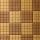How many square tiles with the content 121 cm2 has to be ordered for the paving of the square room with a side length of 2.75 meters?
2. Octagonal mat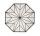Octagonal mat formed from a square plate with a side of 40 cm so that every corner cut the isosceles triangle with leg 3.6 cm. What is the content area of one mat?
3. Areaf of ST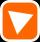It is given square DBLK with side |BL|=13. Calculate area of triangle DKU if vertex U lie on line LB.
4. Square garden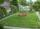On the plan with a scale of 1:1500 is drawn as a square garden with area 81 cm2. How many meters is long garden fence? Determine the actual acreage gardens.
5. Content area and percentsDetermine what percentage is smaller cube surface, when the surface area of the wall decreases by 25%.
6. AceThe length of segment AB is 24 cm and the point M and N divided it into thirds. Calculate the circumference and area of this shape.
7. Chord circleThe circle to the (S, r = 8 cm) are different points A, B connected segment /AB/ = 12 cm. AB mark the middle of S'. Calculate |SS'|. Make the sketch.
8. Right triangleRight triangle ABC with side a = 19 and the area S = 95. Calculate the length of the remaining sides.
9. Right triangle ABCCalculate the perimeter and area of a right triangle ABC, if you know the length of legs 4 cm 5.5 cm and 6.8 cm is hypotenuse.
10. Horizontal distance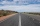The road has a gradient of 8%. How many meters will the road rise on a horizontal distance of 400m?
11. WindbreakA tree at a height of 3 meters broke in the windbreak. Its peak fell 4.5 m from the tree. How tall was the tree?
12. Broken treeThe tree was 35 meters high. The tree broke at a height of 10 m above the ground. Top but does not fall off it refuted on the ground. How far from the base of the tree lay its peak?
13. Sales storesThe first sales store passed the 1/3 and the second 2/5 of the total amount of goods.In third store passed the 2/3 rest of the goods. The remaining 40 kg of goods put into fourth store. How many kilograms of goods pass to a third store?
14. Roses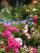On the large rosary was a third white, half red, yellow quarter and six pink. How many roses was in the rosary?
15. ShotsDetermine the percentage rate of keeper interventions if from 32 shots doesn't caught four shots.
16. CandyPeter had a sachet of candy. He wanted to share with his friends. If he gave them 30 candies, he would have 62 candies. If he gave them 40 candies, he would miss 8 candies. How many friends did Peter have?
17. EnrollmentThe enrollment at a local college increased 4% over last year's enrollment of 8548. Find the increase in enrollment (x1) and the current enrollment (x2).